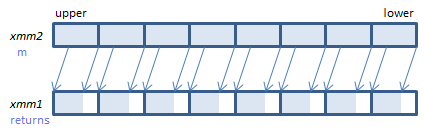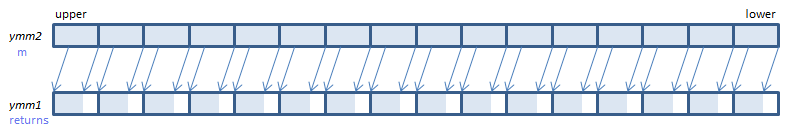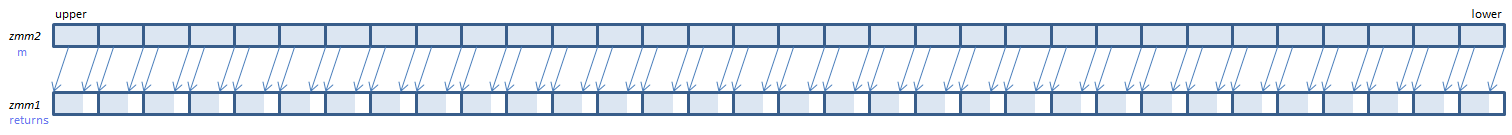﻿ psllw

## PSLLW - Packed Shift Left Logical Word

PSLLW xmm1, imm8    (S2    the number of bits is specified by imm8.
__m128i _mm_slli_epi16(__m128i m, int count)    the number of bits is specified by count.
PSLLW xmm1, xmm2/m128    (S2    the number of bits is specified by the lower QWORD of xmm2/m128.
__m128i _mm_sll_epi16(__m128i m, __m128i count)    the number of bits is specified by the lower QWORD of countFor each WORD, shift the bits to the left by the specified number of bits. Emptied lower bits are zeroed.
VPSLLW xmm1, xmm2, imm8    (V1    the number of bits is specified by imm8.
__m128i _mm_slli_epi16(__m128i m, int count)    the number of bits is specified by count.
VPSLLW xmm1, xmm2, xmm3/m128    (V1    the number of bits is specified by the lower QWORD of xmm3/m128.
__m128i _mm_sll_epi16(__m128i m, __m128i count)    the number of bits is specified by the lower QWORD of count.
VPSLLW xmm1{k1}{z}, xmm2/m128, imm8    (V5+BW+VL    the number of bits is specified by imm8.
__m128i _mm_mask_slli_epi16(__m128i s, __mmask8 k, __m128i m, unsigned int count)    the number of bits is specified by count.
__m128i _mm_maskz_slli_epi16(__mmask8 k, __m128i m, unsigned int count)    the number of bits is specified by count.
VPSLLW xmm1{k1}{z}, xmm2, xmm3/m128    (V5+BW+VL    the number of bits is specified by the lower QWORD of xmm3/m128.
__m128i _mm_mask_sll_epi16(__m128i s, __mmask8 k, __m128i m, __m128i count)    the number of bits is specified by the lower QWORD of count.
__m128i _mm_maskz_sll_epi16(__mmask8 k, __m128i m, __m128i count)    the number of bits is specified by the lower QWORD of count.For each WORD, shift the bits to the left by the specified number of bits. Emptied lower bits are zeroed.
VPSLLW ymm1, ymm2, imm8    (V2    the number of bits is specified by imm8.
__m256i _mm256_slli_epi16(__m256i m, int count)    the number of bits is specified by count.
VPSLLW ymm1, ymm2, xmm3/m128    (V2    the number of bits is specified by the lower QWORD of xmm3/m128.
__m256i _mm256_sll_epi16(__m256i m, __m128i count)    the number of bits is specified by the lower QWORD of count.
VPSLLW ymm1{k1}{z}, ymm2/m256, imm8    (V5+BW+VL    the number of bits is specified by imm8.
__m256i _mm256_mask_slli_epi16(__m256i s, __mmask16 k, __m256i m, unsigned int count)    the number of bits is specified by count.
__m256i _mm256_maskz_slli_epi16(__mmask16 k, __m256i m, unsigned int count)    the number of bits is specified by count.
VPSLLW ymm1{k1}{z}, ymm2, xmm3/m128    (V5+BW+VL    the number of bits is specified by the lower QWORD of xmm3/m128.
__m256i _mm256_mask_sll_epi16(__m256i s, __mmask16 k, __m256i m, __m128i count)    the number of bits is specified by the lower QWORD of count.
__m256i _mm256_maskz_sll_epi16(__mmask16 k, __m256i m, __m128i count)    the number of bits is specified by the lower QWORD of count.For each WORD, shift the bits to the left by the specified number of bits. Emptied lower bits are zeroed.
VPSLLW zmm1{k1}{z}, zmm2/m512, imm8    (V5+BW    the number of bits is specified by imm8.
__m512i _mm512_slli_epi16(__m512i m, unsigned int count)    the number of bits is specified by count.
__m512i _mm512_mask_slli_epi16(__m512i s, __mmask32 k, __m512i m, unsigned int count)    the number of bits is specified by count.
__m512i _mm512_maskz_slli_epi16(__mmask32 k, __m512i m, unsigned int count)    the number of bits is specified by count.
VPSLLW zmm1{k1}{z}, zmm2, xmm3/m128    (V5+BW    the number of bits is specified by the lower QWORD of xmm3/m128.
__m512i _mm512_sll_epi16(__m512i m, __m128i count)    the number of bits is specified by the lower QWORD of count.
__m512i _mm512_mask_sll_epi16(__m512i s, __mmask32 k, __m512i m, __m128i count)    the number of bits is specified by the lower QWORD of count.
__m512i _mm512_maskz_sll_epi16(__mmask32 k, __m512i m, __m128i count)    the number of bits is specified by the lower QWORD of count.For each WORD, shift the bits to the left by the specified number of bits. Emptied lower bits are zeroed.

x86/x64 SIMD Instruction List  Feedback